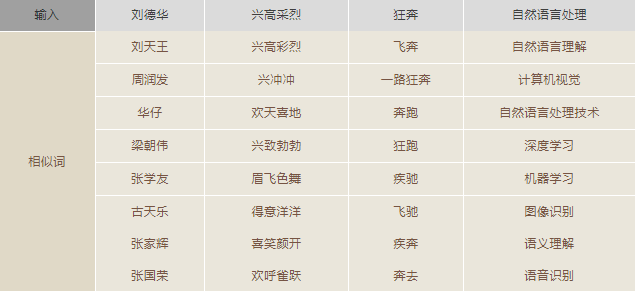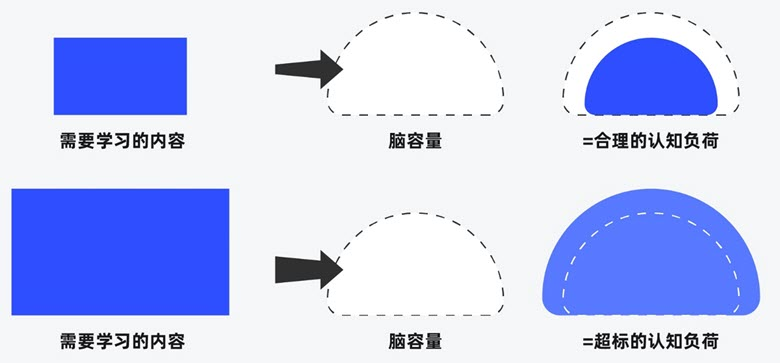# 腾讯AI Lab中文词向量数据使用

## 腾讯AI Lab词向量数据简介### 腾讯AI Lab词向量的特点

• 覆盖率（Coverage）：该词向量数据包含很多现有公开的词向量数据所欠缺的短语，比如“不念僧面念佛面”、“冰火两重天”、“煮酒论英雄”、“皇帝菜”、“喀拉喀什河”等。以“喀拉喀什河”为例，利用腾讯AI Lab词向量计算出的语义相似词如下：墨玉河、和田河、玉龙喀什河、白玉河、喀什河、叶尔羌河、克里雅河、玛纳斯河
• 新鲜度（Freshness）：该数据包含一些最近一两年出现的新词，如“恋与制作人”、“三生三世十里桃花”、“打call”、“十动然拒”、“供给侧改革”、“因吹斯汀”等。以“因吹斯汀”为例，利用腾讯AI Lab词向量计算出的语义相似词如下：一颗赛艇、因吹斯听、城会玩、厉害了word哥、emmmmm、扎心了老铁、神吐槽、可以说是非常爆笑了
• 准确性（Accuracy）：由于采用了更大规模的训练数据和更好的训练算法，所生成的词向量能够更好地表达词之间的语义关系，如下列相似词检索结果所示：### 腾讯AI Lab词向量的构建

• 语料采集：训练词向量的语料来自腾讯新闻和天天快报的新闻语料，以及自行抓取的互联网网页和小说语料。大规模多来源语料的组合，使得所生成的词向量数据能够涵盖多种类型的词汇。而采用新闻数据和最新网页数据对新词建模，也使得词向量数据的新鲜度大为提升。
• 词库构建：除了引入维基百科和百度百科的部分词条之外，还实现了Shi等人于2010年提出的语义扩展算法，可从海量的网页数据中自动发现新词——根据词汇模式和超文本标记模式，在发现新词的同时计算新词之间的语义相似度。
• 训练算法：腾讯AI Lab采用自研的Directional Skip-Gram (DSG)算法作为词向量的训练算法。DSG算法基于广泛采用的词向量训练算法Skip-Gram (SG)，在文本窗口中词对共现关系的基础上，额外考虑了词对的相对位置，以提高词向量语义表示的准确性。

## 腾讯AI Lab词向量的使用

### 词向量数据的加载与使用

# -*- coding:utf-8 -*-
from gensim.models import KeyedVectors

file = 'Tencent_AILab_ChineseEmbedding.txt'
wv_from_text.save('Tencent_AILab_ChineseEmbedding.bin')
print(model.most_similar(positive=['女', '国王'], negative=['男'], topn=1))
print(model.doesnt_match("上海 成都 广州 北京".split(" ")))
print(model.similarity('女人', '男人'))
print(model.most_similar('特朗普', topn=10))
print(model.n_similarity(["中国","北京"],["俄罗斯","莫斯科"]))

Tencent_AILab_ChineseEmbedding读入与高效查询

import numpy as np

embedding_index = {}
f = open(path,encoding='utf8')
for index,line in enumerate(f):
if index == 0:
continue
values = line.split(' ')
word = values
coefs = np.asarray(values[1:],dtype='float32')
embedding_index[word] = coefs
f.close()

return embedding_index

load_embedding('Tencent_AILab_ChineseEmbedding.txt')

ValueError: invalid vector on line 418987 (is this really the text format?)

5 4

wv_from_text = gensim.models.KeyedVectors.load_word2vec_format('Tencent_AILab_ChineseEmbedding_refine.txt',binary=False)

wv_from_text.init_sims(replace=True)  # 神奇，很省内存，可以运算most_similar

### 未知词、短语向量补齐与域内相似词搜索

import numpy as np
def compute_ngrams(word, min_n, max_n):
#BOW, EOW = ('<', '>')  # Used by FastText to attach to all words as prefix and suffix
extended_word =  word
ngrams = []
for ngram_length in range(min_n, min(len(extended_word), max_n) + 1):
for i in range(0, len(extended_word) - ngram_length + 1):
ngrams.append(extended_word[i:i + ngram_length])
return list(set(ngrams))

def wordVec(word,wv_from_text,min_n = 1, max_n = 3):
'''
ngrams_single/ngrams_more,主要是为了当出现oov的情况下,最好先不考虑单字词向量
'''
# 确认词向量维度
word_size = wv_from_text.wv.syn0.shape
# 计算word的ngrams词组
ngrams = compute_ngrams(word,min_n = min_n, max_n = max_n)
# 如果在词典之中，直接返回词向量
if word in wv_from_text.wv.vocab.keys():
return wv_from_text[word]
else:
# 不在词典的情况下
word_vec = np.zeros(word_size, dtype=np.float32)
ngrams_found = 0
ngrams_single = [ng for ng in ngrams if len(ng) == 1]
ngrams_more = [ng for ng in ngrams if len(ng) > 1]
# 先只接受2个单词长度以上的词向量
for ngram in ngrams_more:
if ngram in wv_from_text.wv.vocab.keys():
word_vec += wv_from_text[ngram]
ngrams_found += 1
#print(ngram)
# 如果，没有匹配到，那么最后是考虑单个词向量
if ngrams_found == 0:
for ngram in ngrams_single:
word_vec += wv_from_text[ngram]
ngrams_found += 1
if word_vec.any():
return word_vec / max(1, ngrams_found)
else:
raise KeyError('all ngrams for word %s absent from model' % word)

vec = wordVec('千奇百怪的词向量',wv_from_text,min_n = 1, max_n = 3)  # 词向量获取
wv_from_text.most_similar(positive=[vec], topn=10)    # 相似词查找
compute_ngrams函数是将词条N-grams找出来，譬如：
compute_ngrams('萌萌的哒的',min_n = 1,max_n = 3)>>> ['哒', '的哒的', '萌的', '的哒', '哒的', '萌萌的', '萌的哒', '的', '萌萌', '萌']

### 从词向量文件中提取词语生成自定义词库

from tqdm import tqdm
import re

def gen_dict(input_file, output_file):
output_f = open(output_file, 'a', encoding='utf8')
with open(input_file, "r", encoding='utf-8') as f:
for i in tqdm(range(vocab_size)):
word = line.split(' ')
output_f.write(word + '\n')
output_f.close()
f.close()

def gen_dict_with_chinese(input_file, output_file):
pattern = re.compile("[\u4e00-\u9fa5]")
output_f = open(output_file, 'a', encoding='utf8')
with open(input_file, "r", encoding='utf-8') as f:
for line in tqdm(lines):
line = line.strip()
if re.findall(pattern, line):
output_f.write(line + "\n")
output_f.close()

def gen_dict_small(input_file, output_file, size=10000):
output_f = open(output_file, 'a', encoding='utf8')
with open(input_file, "r", encoding='utf-8') as f:
output_f.write(str(size) + " 200\n")
for i in tqdm(range(size)):
output_f.write(line)
output_f.close()
f.close()

if __name__ == "__main__":
# gen_dict('./Tencent_AILab_ChineseEmbedding.txt',
#          './dict.txt')
# gen_dict_with_chinese('./dict.txt', './dict-no-alnum.txt')
gen_dict_small(r'D:\ProgramData\Tencent_AILab_ChineseEmbedding.txt', 'dict_10000.txt', 10000)##### 界面设计和游戏设计中的认知负荷##### 理解认知偏差